I use the Open Source photo management Software Digikam (along with other tools such as Gimp and DarkTable).  I obviously need very little encouragement to combine my geeky hobbies, so I quickly tried to interrogate Digikam with R, which is easy, because Digikam keeps all it's image info in a SQLite database, which R has support for.

So this post shows how I did it, along with some of the output, such as the focal length of my images over time, looks like I need a telephoto lens !  (this script and my digikam db are in github here)

``````
ggplot(data = subset(ImgMerge, model == "NIKON D5000" & focalLength < 60), aes(x = as.POSIXct(creationDate),
y = focalLength)) + geom_point(alpha = 0.2)
``````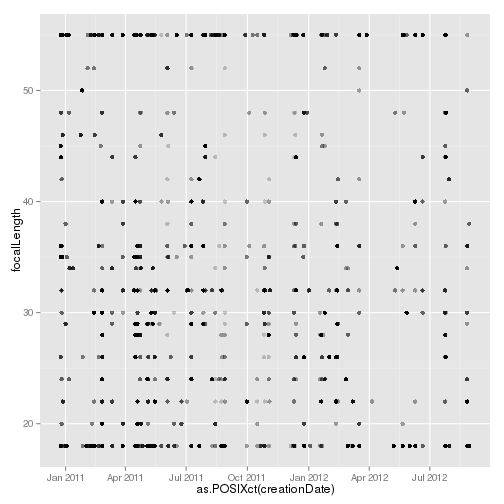``````
library(RSQLite)
``````
``````## Loading required package: DBI
``````

`library(ggplot2) library(plyr) m <- dbDriver("SQLite") basedir <- "/home/paul/RStudio/DigikamR/" con <- dbConnect(m, dbname = paste(basedir, "data/digikam4.db", sep = "")) `

Now we've opened the database, we can examine some of the tables within it.

``````
# List the tables in the database
dbListTables(con)
``````
``````##   "AlbumRoots"         "Albums"             "DownloadHistory"
##  "
ImagePositions"     "ImageProperties"    "ImageRelations"
##  "ImageTagProperties" "ImageTags"          "Images"
##  "Searches"           "Settings"           "TagProperties"
##  "Tags"               "TagsTree"
``````
``````
# List the columns of some of the interesting tables
``````
``````##   "imageid"          "rating"           "creationDate"
##   "digitizationDate" "orientation"      "width"
##   "height"           "format"           "colorDepth"
##  "colorModel"
``````
``````names(dbReadTable(con, "ImageComments"))
``````
``````##  "id"       "imageid"  "type"     "language" "author"   "date"
##  "comment"
``````
``````names(dbReadTable(con, "ImageMetadata"))
``````
``````##   "imageid"                      "make"
##   "model"                        "lens"
##   "aperture"                     "focalLength"
##   "focalLength35"                "exposureTime"
##   "exposureProgram"              "exposureMode"
##  "sensitivity"                  "flash"
##  "whiteBalance"                 "whiteBalanceColorTemperature"
##  "meteringMode"                 "subjectDistance"
##  "subjectDistanceCategory"
``````
``````names(dbReadTable(con, "ImageProperties"))
``````
``````##  "imageid"  "property" "value"
``````
``````names(dbReadTable(con, "ImagePositions"))
``````
``````##   "imageid"         "latitude"        "latitudeNumber"
##   "longitude"       "longitudeNumber" "altitude"
##   "orientation"     "tilt"            "roll"
##  "accuracy"        "description"
``````
``````names(dbReadTable(con, "Images"))
``````
``````##  "id"               "album"            "name"
##  "status"           "category"         "modificationDate"
##  "fileSize"         "uniqueHash"
``````
``````names(dbReadTable(con, "TagProperties"))
``````
``````##  "tagid"    "property" "value"
``````

And now we can pull some of the inetresting tables into a dataframe

``````
# Pull some of the information together
# and merge it together
ImgMerge <- merge(Imgs, ImgMeta, by.x = "id", by.y = "imageid")
ImgMerge <- merge(ImgMerge, ImgInfo, by.x = "id", by.y = "imageid")
# clean it up
ImgMerge\$make <- as.factor(ImgMerge\$make)
ImgMerge\$model <- as.factor(ImgMerge\$model)
ImgMerge\$faperture <- as.factor(ImgMerge\$aperture)
ImgMerge\$fexposureTime <- as.factor(ImgMerge\$exposureTime)
ImgMerge\$fmodel <- as.factor(ImgMerge\$model)
ImgMerge\$Year <- format(as.POSIXct(ImgMerge\$creationDate), format = "%Y")
ImgMerge\$Month <- format(as.POSIXct(ImgMerge\$creationDate), format = "%b")
``````

Here are some plots

``````# and draw some graphs
ggplot(data = subset(ImgMerge, focalLength < 60), aes(x = as.POSIXct(creationDate),
y = focalLength, colour = model)) + geom_point()
``````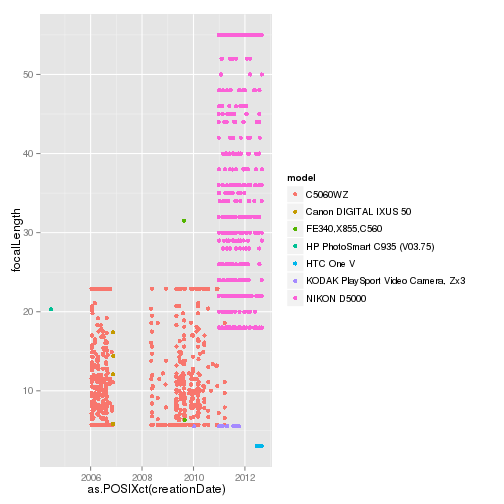``````
ggplot(data = ImgMerge, aes(x = focalLength)) + geom_histogram(binwidth = 5,
aes(colour = as.factor(model))) + facet_grid(model ~ .)
``````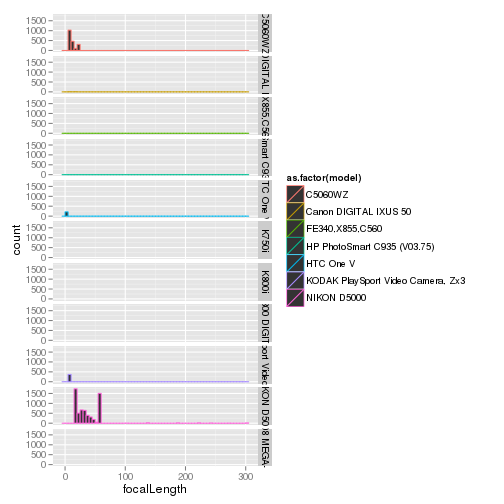``````
qplot(data = ImgMerge, x = as.numeric(as.character(aperture)), y = log(as.numeric(as.character(exposureTime))),
colour = as.factor(model), geom = "point")
``````
``````## Warning: Removed 2638 rows containing missing values (geom_point).
``````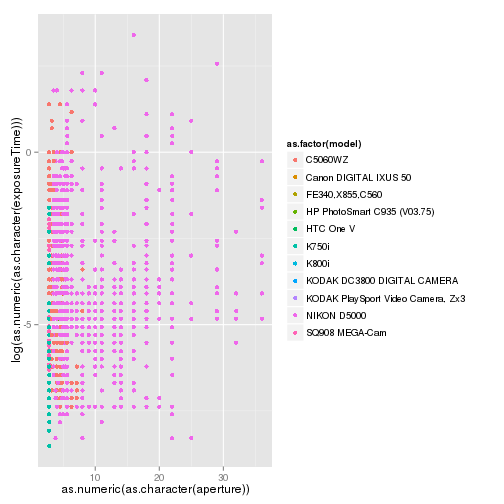``````
ggplot(data = subset(ImgMerge, model == "NIKON D5000"), aes(x = focalLength)) +
geom_histogram(binwidth = 5) + facet_grid(Year ~ .)
``````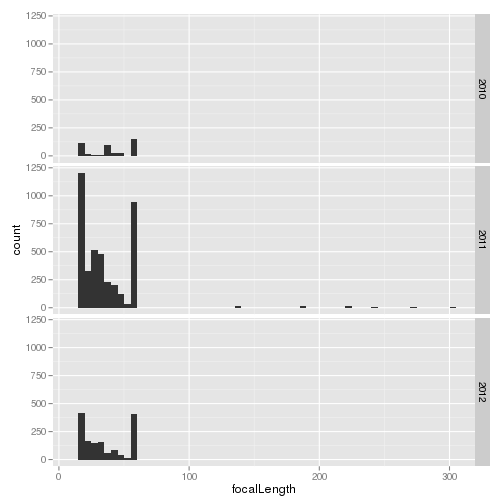``````
ggplot(data = subset(ImgMerge, model == "NIKON D5000"), aes(x = as.POSIXct(creationDate),
y = focalLength)) + geom_point()
``````
``````## Warning: Removed 14 rows containing missing values (geom_point).
``````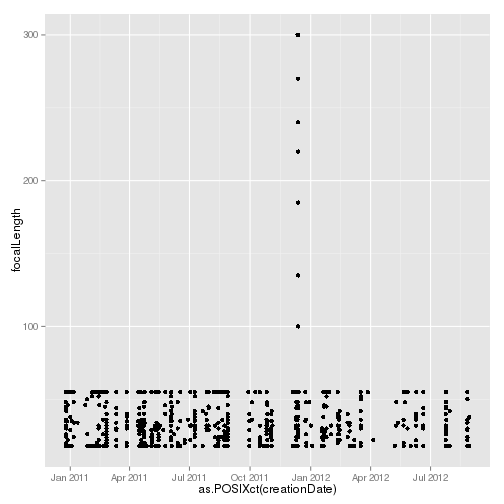``````
ggplot(data = subset(ImgMerge, model == "NIKON D5000" & focalLength < 60), aes(x = as.POSIXct(creationDate),
y = focalLength)) + geom_point(alpha = 0.2)
``````